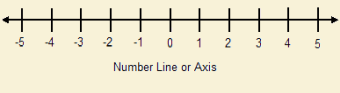# A Negative Times a Negative Makes a Positive Number

1. Home
2. /
3. Mathematics
4. /
5. A Negative Times a...In high school we were introduced to negative numbers. Why high school? Why not earlier? Because we cannot picture in our minds what a negative number is. We know what positive numbers are. For instance, if we have three apples and someone gives us four more apples, we know we now have seven apples. And as to multiplication, if we have three groups of four three apples each, we know we have 12 apples. But can you visualize what a negative apple might be? How can you demonstrate negative times negative makes positive?

## Pure Numbers

Forget units for the moment. We will concentrate on pure numbers. In the above example, the four groups of three apples becomes simply 4(3) = 12. Suppose, instead of 4(3) we make on of the numbers negative. Let’s use –3. We now have

4(–3) = ?

We could be a dead horse, but it seems pretty intuitive that four groups of –3 should add up to –12.

But what if both numbers are negative. Do we wind up with a positive number? We write

(–4)(–3) = ?

## Modifying the Equation

Our goal is not to give a rigorous mathematical proof, but to provide intuitive insight concerning negative numbers. Let’s modify the equation to read

–4(–3 + 3) = ?

This equation suggests the equivalence of subtracting a positive number to adding a negative one. That being the case, we see

–4(–3 + 3) = –4(–3) + –4(3) = 0

–4(–3) + (–12) = 0

Or, moving the –12 to the other side of the equals sign,

–4(–3) = +12

## Negative times negative makes positive

We see from the above that negative four times negative three makes positive twelve. In using pure numbers instead of numbers with units, we took away the stumbling block of preconceived mental prejudice.

Note: You might also enjoy What is a Negative Number?

### 2 thoughts on “A Negative Times a Negative Makes a Positive Number”

• Funnily enough, I never had any problem with this, maybe I didn’t know enough to question it at the time, apart from the old saying, “Two wrongs don’t make a right”!

• I figure few did have a problem with it. That’s the thing with young people. They are quick to “buy into” what they are being taught. This is a good thing and a bad thing, both. But it is important to either prove or establish beyond reasonable doubt that a matter is true before building on it. Besides, it’s in us to solve such puzzles, isn’t it?